# Merge K Sorted Lists (With C++, Java and Python Code)

Tech Companies like to hire candidates with good problem-solving capabilities. So, they come up with a variety of problems to assess their skills. These problems are nothing but variations of some major and well-known problems. One such problem is the “merge k sorted lists” problem. In this article, we will discuss this problem and the approaches to solving this problem, with their pros, cons, and code. So, get ready because it is going to be an interesting ride!

## Problem Statement

“Given k linked lists, each linked list is sorted in ascending order. Merge all the linked lists into one sorted linked list and return it.”

Look at the illustration below to understand the problem statement better.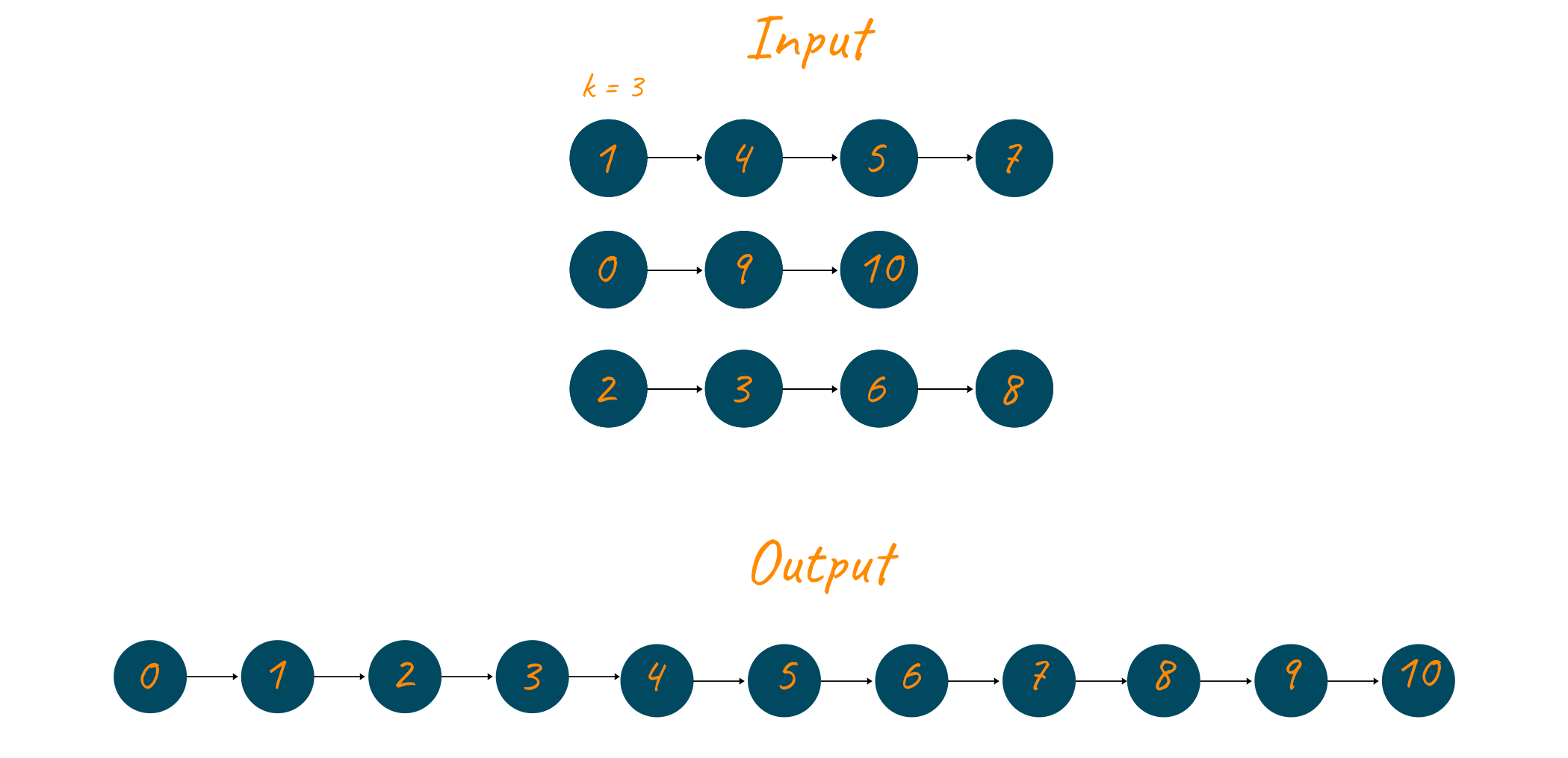## Solutions for Merge k Sorted List Problem

Several approaches exist in the programming world to solve this problem, some efficient and some not so efficient. Let us build the intuition to solve this problem brick by brick, by first discussing some simple methods, which are not so efficient.

The first way of solving this problem is by connecting all the k linked lists into one list, ignoring the order of the elements at this step. After this use some sorting algorithm, like merge sort to sort the list in the ascending order. That’s it! the problem is solved. However, this approach is not so efficient as it does not take the advantage of the fact that each linked list is already sorted. This method has the time complexity of O(n.log(n)).

The second naïve approach to solve this problem is by making the first list the result list. Then traverse through each of the remaining lists, starting from the second list, and insert every node of the currently traversed list into the result list at its correct position, such that the resulting result list is sorted. This method is also very simple, right? But this method has a high time complexity of O(nk2). Where n is the total number of elements across all the lists.

From the above two approaches, we saw that we need a method that takes advantage of the lists being sorted and generates a new sorted list efficiently. What if we use some data structure that is specifically designed to give us the min element at each step, Does a data structure like this exists? Well, the answer is yes. There is a data structure called the min-heap, which will help us in solving this problem. If you are not familiar with the min-heap data structure, then we highly recommend you to go through this article first before continuing.

## Merging K Sorted Lists using Min Heap

The idea here is to take advantage of the fact that the root of a min-heap always gives us the smallest element. What we do in this approach is that since all the linked lists are already sorted, therefore the first elements of all of the lists will be the smallest element in their respective lists. We take advantage of this situation by putting the first element of all the linked lists into a min-heap. We then extract the top element (root) of the min-heap to get the smallest element. By doing this we get the smallest element across all the linked lists. After this, we increment the pointer of the list to which the recently extracted element belonged to point to the next element, and that element is now added to the min-heap. An element from the min-heap is again extracted, the pointer of the linked list which contained that element is incremented and the newly pointed element is added to the min-heap. This process continues until the min-heap is empty. Note that we are maintaining a separate result list, to which keep adding the element which we extract from the min-heap.

A major advantage of this method is its adaptability. This method can be appointed to merge K sorted arrays as well, with some small tweaks here and there. This method works well even when all the linked lists are not of the same size, which some of the other methods fail to achieve.

### Algorithm

Let us first look at the algorithm of this approach and then we will understand it with the help of an example.

1) Construct a min-heap and put the first element of each of the 'k' linked lists in it.

2) Perform the following steps until the min-heap is not empty:

2.1) Remove the root element of the min-heap and add it to the result list

2.2) If an element (in the same linked list) exists adjacent to the element that was popped out in the previous phase, add it to the min-heap.

### Example

Let us take an example now to better understand the algorithm. Suppose we are given the following linked lists.Now the min-heap is built using the first element of all the linked lists. See below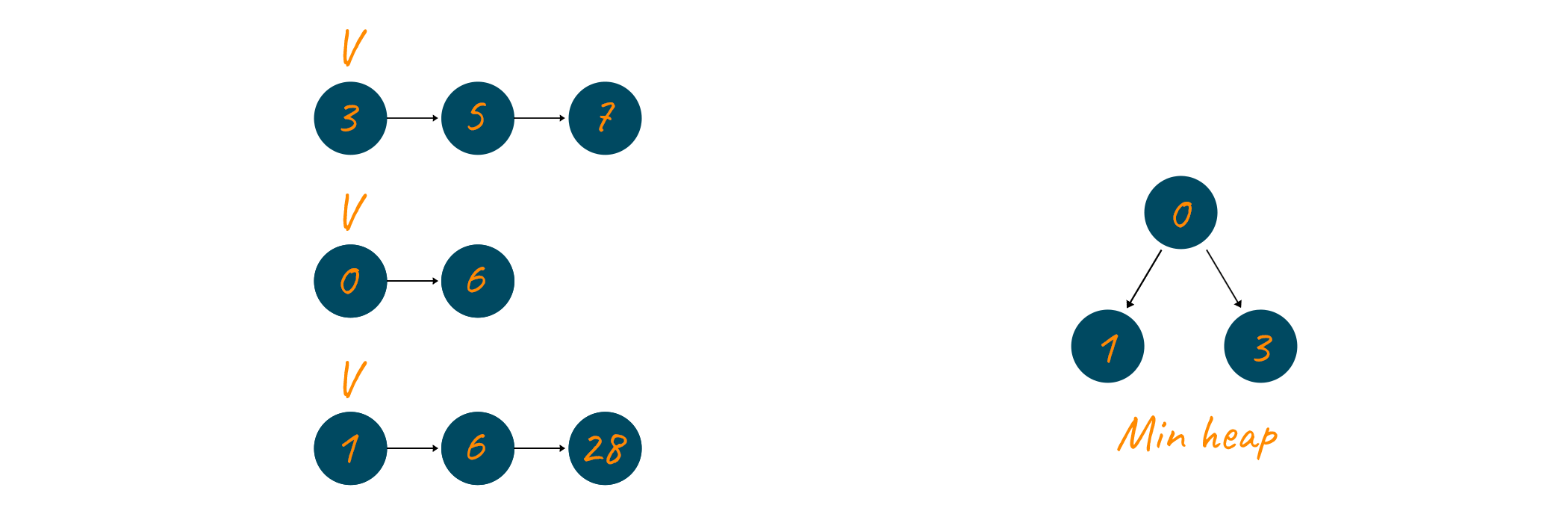The root element (0) of this min-heap is popped. The element popped belongs to the second linked list and since it contains an element (6) next to it, therefore the pointer of the second linked list is updated to point to 6 now and 6 is inserted into the min-heap. The element extracted at this step, which is 0, is inserted into the result list.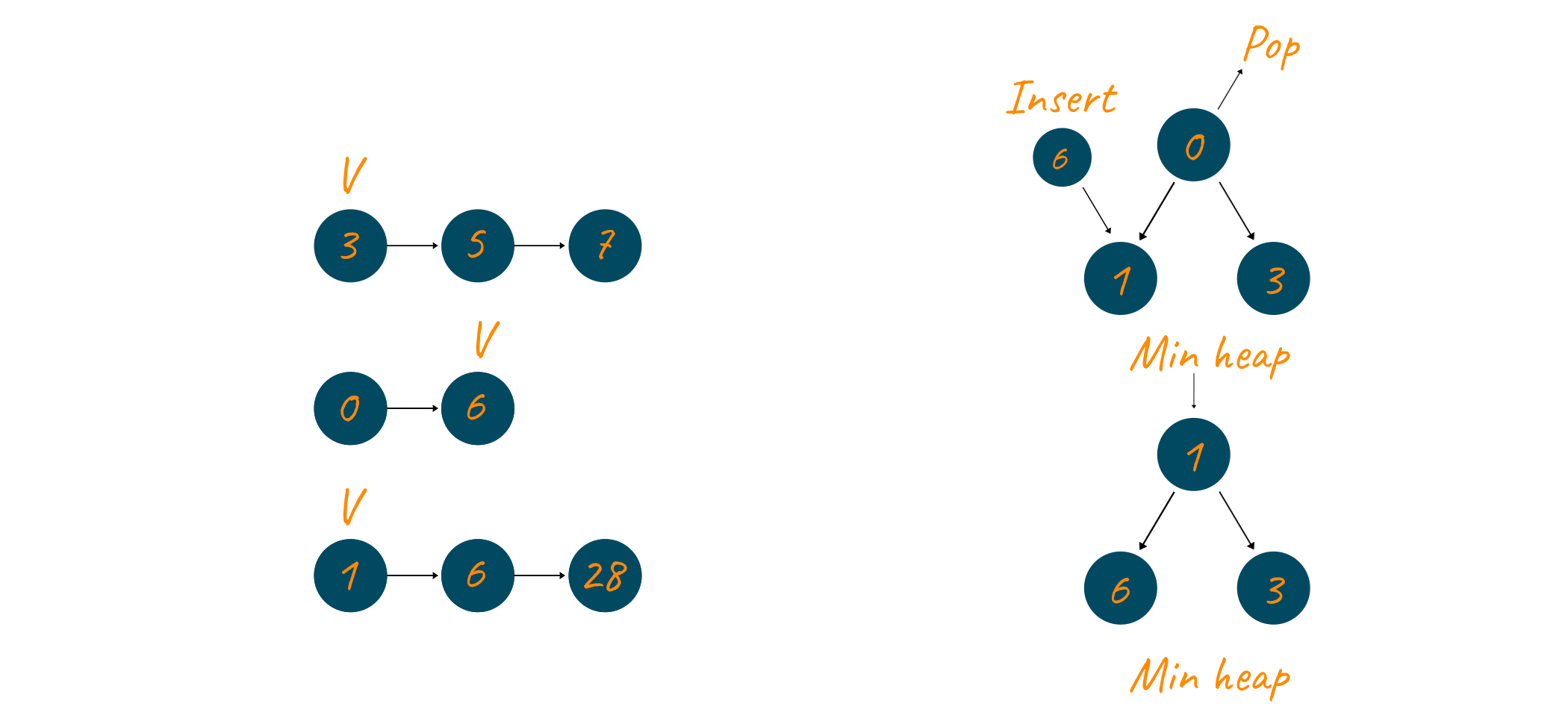The root element i.e., 1 is again popped and added to the result linked list. Since 1 belonged to the third linked list/, therefore, the pointer of this linked list is updated to point to the next element, which is 6 and it is added to the min-heap.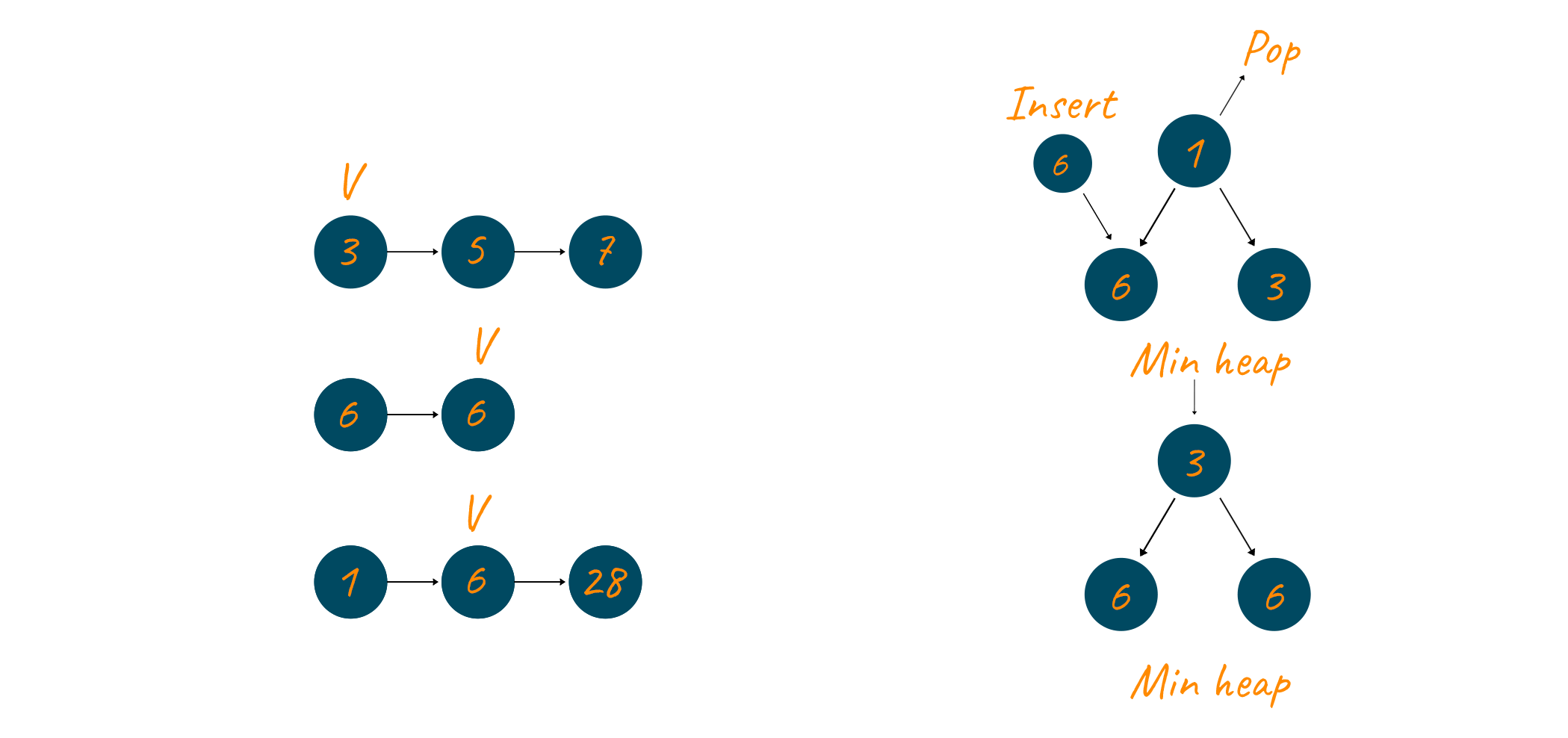Now, 3 is now extracted from the min-heap and inserted into the result linked list. The pointer of the first linked list is updated to now point to the next element (5) and 5 is added to the min-heap.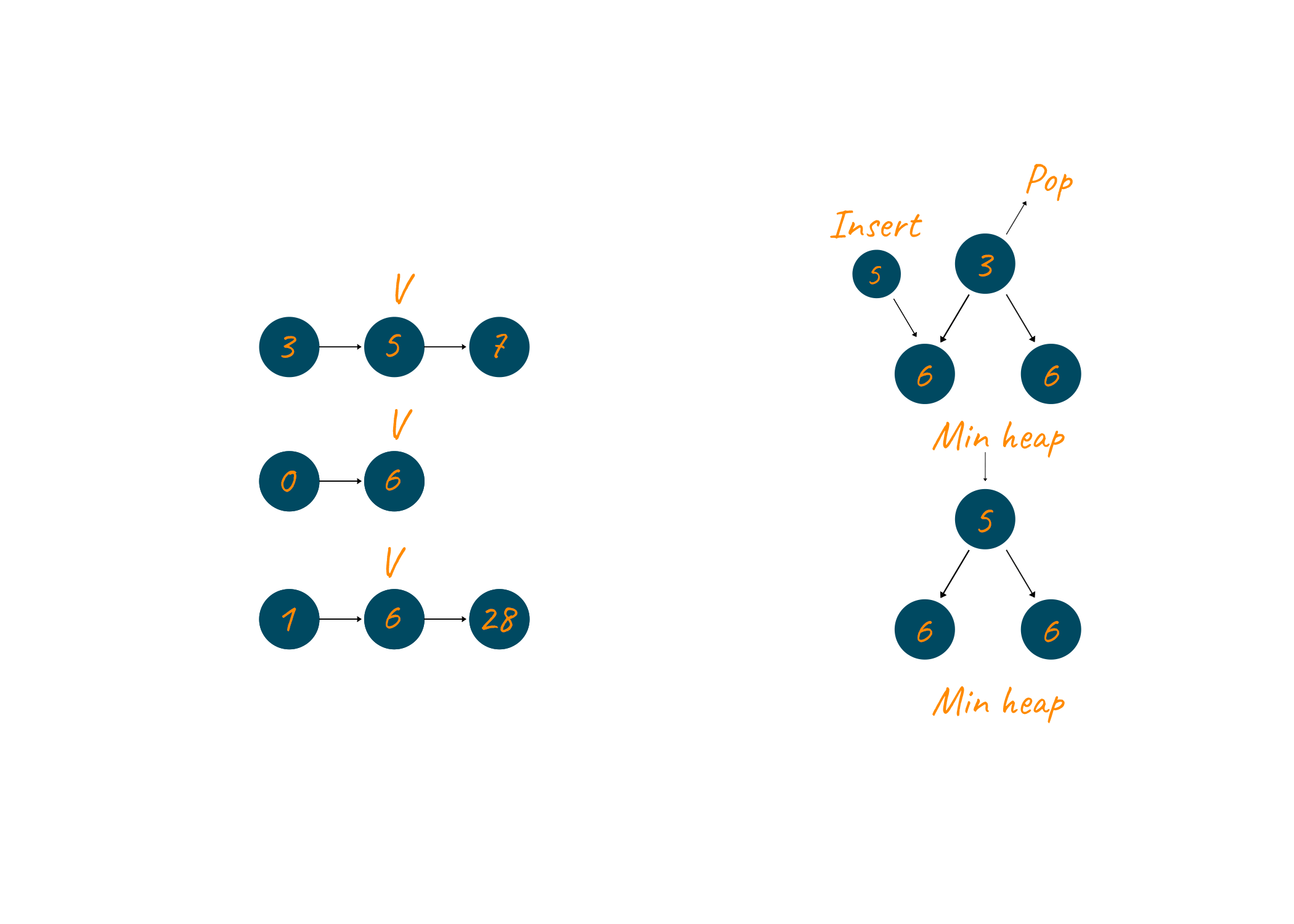The number 5 is now extracted and it is added to the result linked list. Since 5 belonged to the first linked list therefore its pointer is updated to point to the next element (7) and this element is added to the min-heap.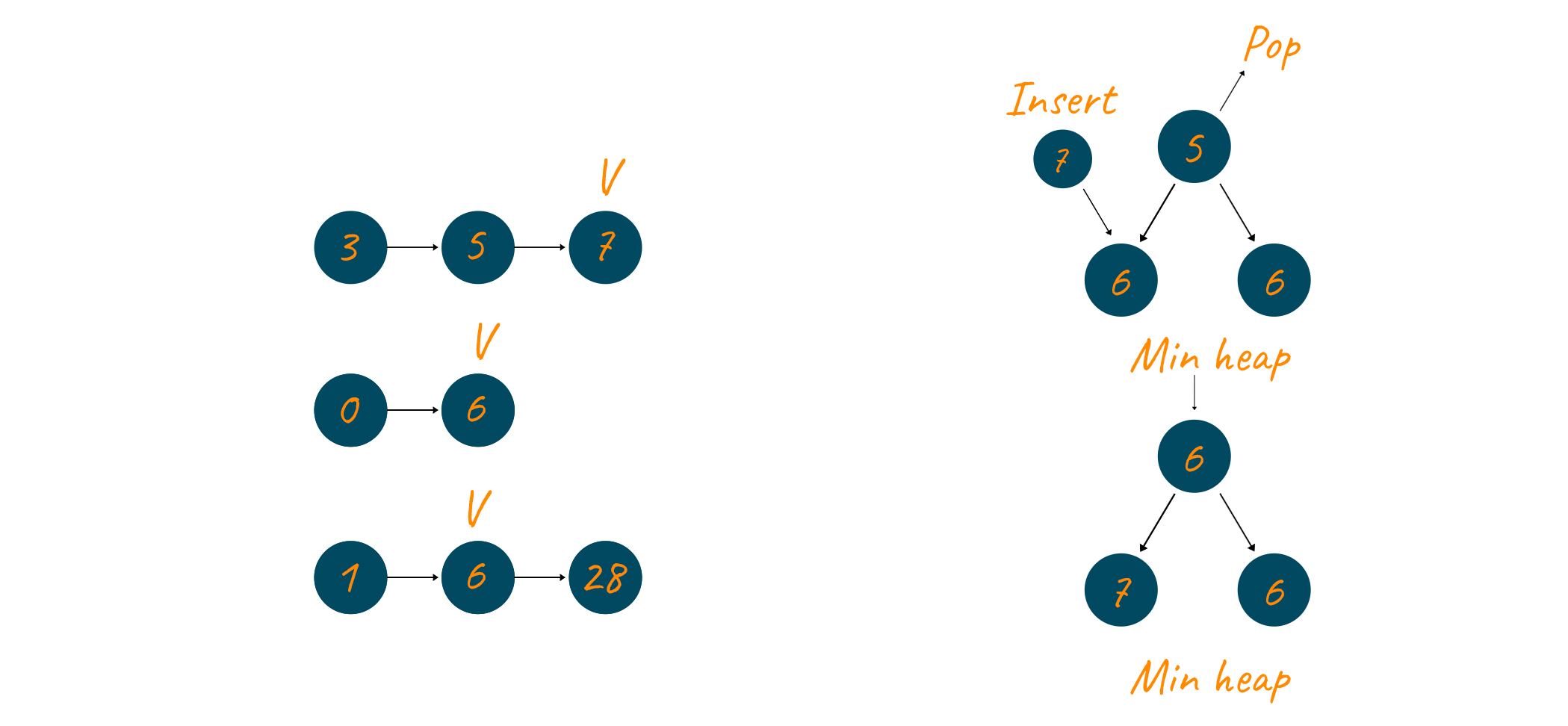The root node, 6, is again extracted and added to the min-heap. The second linked list’s pointer is updated since no element is present in this linked list therefore no element is added to the min-heap at this step.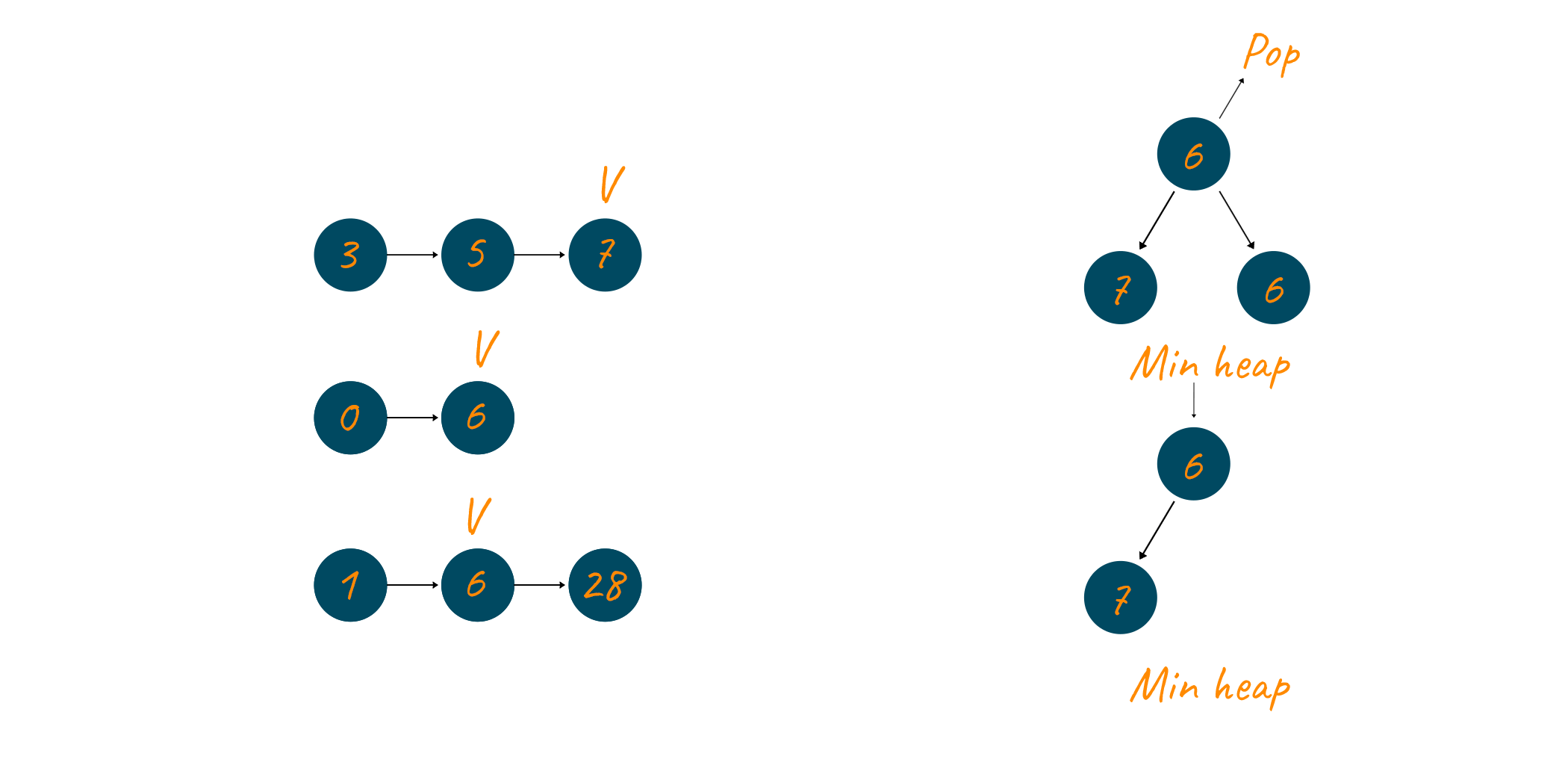The number 6, this time from the third linked list is removed from the min-heap. The pointer of the third linked list is updated and the number 28 is added to the min-heap.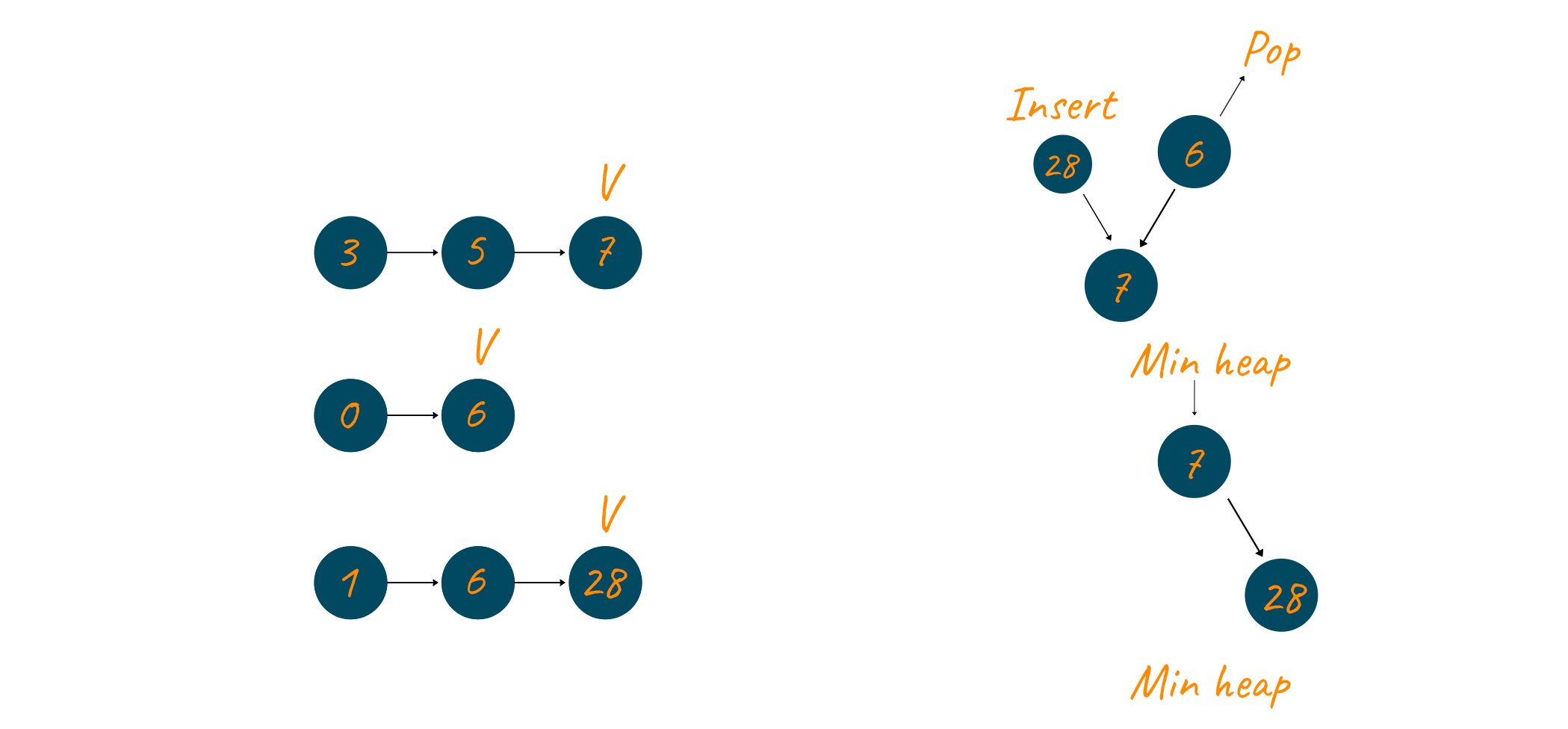This time the number 7 is popped and the pointer of the first linked list is updated. Since this time also there are no more elements left in the first linked list, therefore, no element is inserted into the min-heap.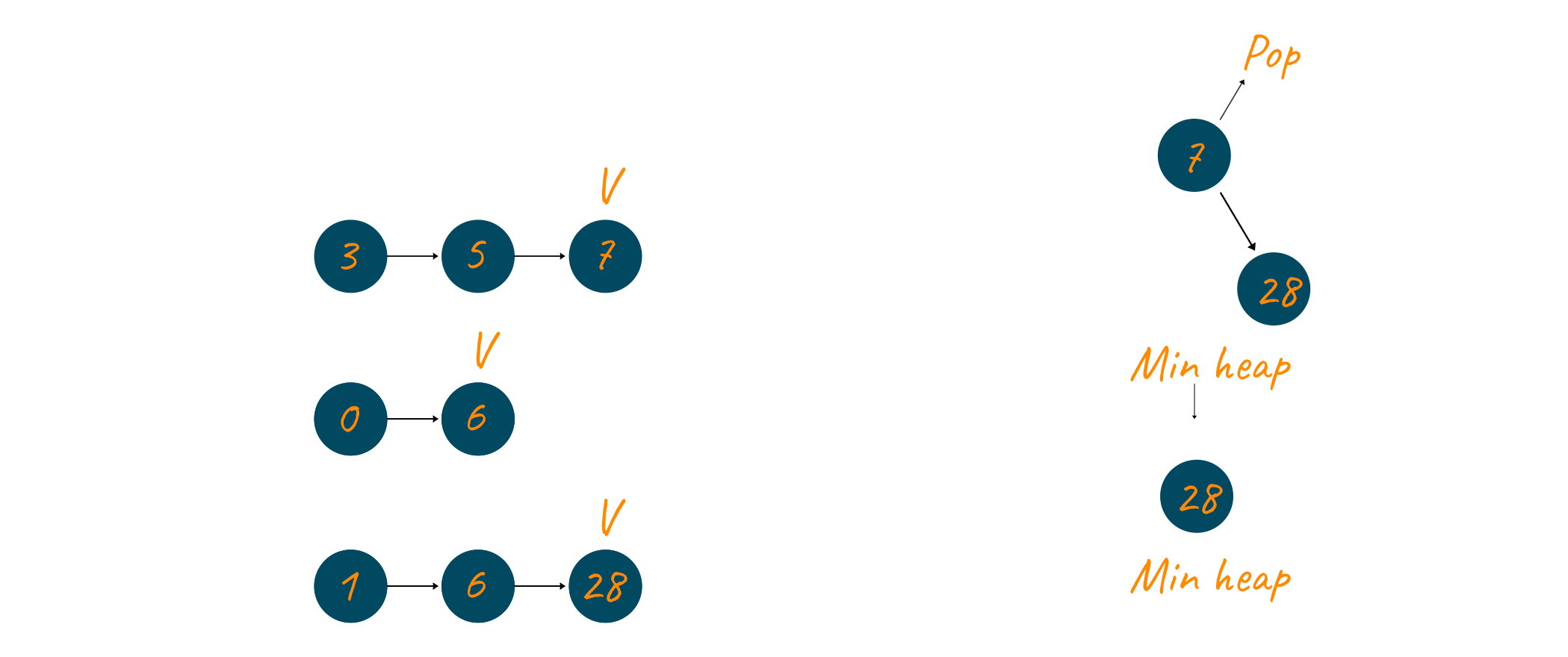Now only one element is left in the min-heap and that element, 28, is extracted. The pointer of the third linked list is updated and because no more elements are present in the linked list that is why none are inserted.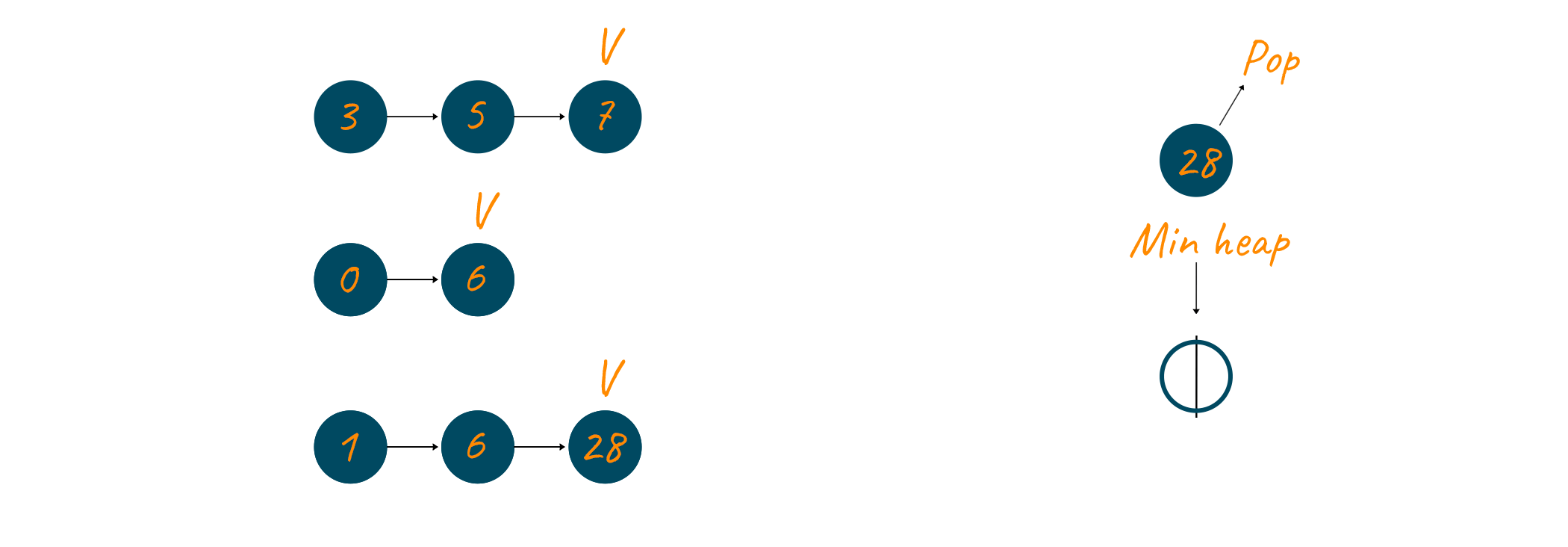At this point the min-heap is empty and we get the result linked list as our merged linked list. At the end of this algorithm, the result linked list looks like this.### Codes in different programming languages

Let us now look at the code of this algorithm in Java, C++, and python-

#### 1) Java Code

```import java.util.PriorityQueue;

class Node{
int data;
Node next;
Node(int val){
data=val;
next=null;
}
}

public class mergeKSortedList {

//function to merge k sorted linked lists.
static public Node merge(Node[] arr,int k){

//PriorityQueue 'pq' implemented as min heap using lambda expression
PriorityQueue<Node> pq=new PriorityQueue<>((a,b)->{
return a.data-b.data;
});

//Pushing the head nodes of all
//the k lists in 'pq'
for(int i=0;i<k;i++){
if(arr[i]!=null){
}
}
//Handling the case when k=0 or lists are empty.
if(pq.isEmpty()) return null;

//Creating dummy node
Node dummy=new Node(-1);
Node last=dummy;

//Run while loop till priorityQueue(pq) is not empty
while(!pq.isEmpty()){
//Get the top element of 'pq'
//which is the minimum of all existing nodes.
Node curr=pq.remove();

// to the resultant merged list
last.next=curr;
last=last.next;

// Check if there is a node
// next to the 'top' node
// in the list of which 'top'
// node is a member
if(curr.next!=null){
}
}
//return the next of dummy node
// which the actual head of merged list.
return dummy.next;
}

//function to print the sorted linked list
}
System.out.print("none");
}

public static void main(String[] args) {
//Number of linked lists to be merged.
int k=3;
Node[] arr=new Node[k];

arr=new Node(3);
arr.next=new Node(5);
arr.next.next=new Node(7);

arr=new Node(0);
arr.next=new Node(6);

arr=new Node(1);
arr.next=new Node(6);
arr.next.next=new Node(28);

//Calling the merge function to merge all the linked lists
}

}
```

#### 2) C++ Code

```#include <bits/stdc++.h>
using namespace std;

struct Node {
int data;
struct Node* next;
};

struct Node* newNode(int data) {
struct Node* new_node = new Node();
new_node->data = data;
new_node->next = NULL;

return new_node;
}

// 'compare' function used to build
// up the priority queue
struct compare {
bool operator()(
struct Node* a, struct Node* b) {
return a->data > b->data;
}
};

// Function to merge k sorted linked lists
struct Node* mergeKSortedLists(
struct Node* arr[], int k)
{
// Priority_queue 'pq' implemented
// as min heap with the help of
// 'compare' function
priority_queue<Node*, vector<Node*>, compare> pq;

// Push the head nodes of all
// the k lists in 'pq'
for (int i = 0; i < k; i++)
if (arr[i] != NULL)
pq.push(arr[i]);

// Handles the case when k = 0
// or lists have no elements in them
if (pq.empty())
return NULL;

struct Node *dummy = newNode(0);
struct Node *last = dummy;

// Loop till 'pq' is not empty
while (!pq.empty()) {
// Get the top element of 'pq'
struct Node* curr = pq.top();
pq.pop();

// Add the top element of 'pq'
// to the resultant merged list
last->next = curr;
last = last->next;

// Check if there is a node
// next to the 'top' node
// in the list of which 'top'
// node is a member
if (curr->next != NULL)

// Push the next node of top node
// in 'pq'
pq.push(curr->next);
}

return dummy->next;
}

// Function to print the linked list
cout << head->data << " ";
}
}

int main() {
int k = 3;
// Number of elements in each list
int n = 4;

// An array of pointers storing the head nodes of the linked lists
Node* arr[k];

arr = newNode(1);
arr->next = newNode(3);
arr->next->next = newNode(5);
arr->next->next->next = newNode(7);

arr = newNode(2);
arr->next = newNode(4);
arr->next->next = newNode(6);
arr->next->next->next = newNode(8);

arr = newNode(0);
arr->next = newNode(9);
arr->next->next = newNode(10);
arr->next->next->next = newNode(11);

struct Node* head = mergeKSortedLists(arr, k);

return 0;
}
```

#### 3) Python Code

```import heapq
from heapq import heappop, heappush

class Node:
def __init__(self, data, next=None):
self.data = data
self.next = next

def __lt__(self, other):
return self.data < other.data

# function to print contents of a linked list
def printList(node):
while node:
print(node.data, end=' —> ')
node = node.next

print('None')

def mergeKLists(lists):

# create a min-heap using the first node of each list
pq = [x for x in lists]
heapq.heapify(pq)

while pq:

# extract node from the min-heap
min = heappop(pq)

# add the minimum node to the output list
last = min
else:
last.next = min
last = min

# take the next node from the "same" list and insert it into the min-heap
if min.next:
heappush(pq, min.next)

# return head node of the merged list

k = 3

lists = [None] * k

lists = Node(3)
lists.next = Node(5)
lists.next.next = Node(7)

lists = Node(0)
lists.next = Node(6)

lists = Node(1)
lists.next = Node(6)
lists.next.next = Node(28)

# Merge all lists

```

Output:

```0 —> 1 —> 3 —> 5 —> 6 —> 6 —> 7 —> 28 —> None
```

### Time complexity

This method has a time complexity of O(n.log(k)). Where n is the total number of elements across all the lists and k is the number of linked lists in the question. Let now discuss how is this complexity is calculated.

A min-heap is essentially a complete binary tree and a complete binary tree has 1 + floor( log x) levels. Where x is the total number of elements in the tree. Since in this algorithm the min-heap can have at max k element at any given instant, therefore, x is equal to k here. So, in this case, we are going to have 1+ floor(log k) levels, which is O(log k). Now for all the elements (n), we are going to perform insertion in the binary tree. The cost insertion for an element in a heap is equal to the number of levels in the heap. Since the number of levels is log k in this case, therefore the cost of insertion per element is log k. Now for adding n elements the total cost will be n log k.

Let us now come to the removal of elements. Removal also takes the same time as insertion in a binary heap, therefore, the time required to remove n elements from the binary heap is n log k. To get a better intuition of the time complexity calculation for insertion and removal of elements in a binary heap, refer to this article. Now the total time to remove and insert n elements in a binary heap is equal to 2 n log k. Therefore,  the time complexity of this algorithm calculates to be O(n.log(k))

### Space Complexity

This method has a space complexity of O(K). Let us now discuss why is this so. At any instant of time, the min-heap can have at max k elements. Why? Because at any instant the min-heap is going to store the smallest item of every list. There are k lists and therefore the space complexity is O(k).

## Conclusion

This problem tests a candidate's problem-solving skills and his or her grasp on the concepts of linked list and heap. We highly encourage you to have a good grasp on major data structures. Also, if you need help with your data structures assignments then our experts are available 24/7.  In this article, we first discussed the problem statement. We then discussed two inefficient approaches to solving this problem, followed by an efficient approach. We, at last, had a detailed discussion about the time and space complexity of the efficient approach. When it comes to such types of problems, solving them is not only the key but solving the problem efficiently is crucial.

### FavTutor - 24x7 Live Coding Help from Expert Tutors!